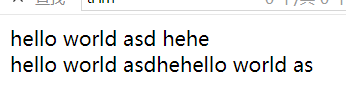```//创建数组
\$a = array(
"a"=>1,
"b"=>12,
"age"=>31,
"a"=>22
);
\$b = array(
"age"=>1
);
\$c = array(
1,
"age"=>12
);
//比较多个数组值的差异 返回\$a中存在 但后边元素集合中不存在的值
print_r(array_diff(\$a,\$b,\$c));
//返回数组的交集
print_r(array_intersect(\$a,\$b,\$c));
//返回数组的键的集合
print_r(array_keys(\$a));
//返回数组的值的集合
print_r(array_values(\$a));
//去除重复的键 在原来的数组上改变 而且返回值为操作的数组
print_r(array_unique(\$a));

//添加多个元素 进栈
array_push(\$a,1,2);
//出栈
array_pop(\$a);//只能出栈一个元素 只能传递数组出栈最后一个元素
print_r(\$a);
```

```echo "<meta charset=utf8>";
\$sum = array(1,2,3,4,5);
function sum(\$value,\$key,\$p){
//\$sum[\$key]+=1;
echo \$key;
echo \$value;
echo \$p;
}
array_walk(\$sum,"sum","这是一个参数");
print_r(\$sum);//对数组中的每个元素都做回调处理
```

php处理字符串的常见函数重点关注参数和返回值

剔除字符

```/*第二个参数为空时 默认\0 \t \n \x0B \r 都会被剔除*/
\$str = " hello world asd\n";
\$html = "   hehe";
echo trim(\$str).\$html."<br>";
echo trim(\$str).trim(\$html);
echo trim(\$str," hed\n");//第二个参数只能是字符串的开头或者结尾开始匹配并且剔除不能直接从中间开始 还有ltrim rtrim``````/*第二个参数为空时 默认\0 \t \n \x0B \r 都会被剔除*/
\$str = " hello world asd\n";
echo strlen(\$str);
/*

mb_strlen并不是PHP核心函数，使用前需要确保在php.ini中加载了php_mbstring.dll，即确保

“extension=php_mbstring.dll”这一行存在并且没有被注释掉，否则会出现未定义函 数的问题。
*/
echo mb_strlen("我是中国人",'UTF8');
```

subStr函数

`echo "<br>".substr("I am your father!!!",2,2);//substr(string,start,length)从第2个开始截取两位 应该为am`

str_replace

```\$test = "I am your father";
echo str_replace("father","mother",\$test);//将father替换成mother```

str_repeat和is_string

```echo str_repeat("-",10);//重复字符串
\$a = 1;
print(is_string("asdf"));//判断是否为字符串
```

sprintf返回格式化后的字符串

```\$txt = sprintf("%s 每天有 %u 万人在访问！", \$str, \$number);
```